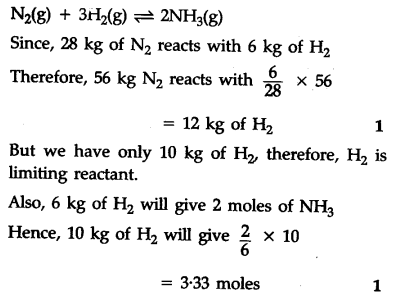# 56 kg of \${{N}_{2}}\$(g) and 10 kg of \${{H}_{2}}\$(g) are mixed to produce N\${{H}_{3}}\$(g)

56 kg of \${{N}{2}}\$(g) and 10 kg of \${{H}{2}}\$(g) are mixed to produce N\${{H}_{3}}\$(g). Calculate the number of moles of ammonia gas formed.
Atomic mass/g \${{m}^{-1}}\$ N = 14, H = 1)